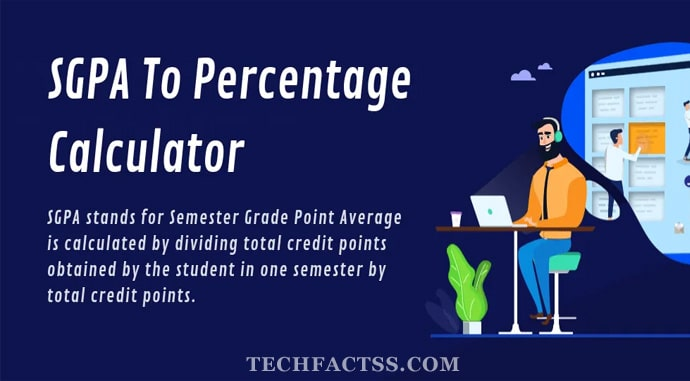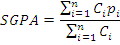# SGPA To Percentage Calculator | Convert SGPA to Percentage

SGPA To Percentage Calculator: If you are a student with any reputed university or college, you are aware of the concept called SGPA. It forms part of the grading system that is used for analyzing the performance of a student in the examination. The SGPA performance is applied to the students appearing for the semester examinations. But then, most of you would be looking to convert SGPA to percentage. It can be a little confusing, but you can use a few options to convert SGPA to percentage.

SGPA stands for Semester Grade Point Average is calculated by dividing total credit points obtained in one semester by total credit points.## How to Convert SGPA into Percentage?

SGPA stands for Semester Grade Point Average. It is a sheer number that indicates the performance of a student in the semester examinations. It includes the weighted average of the marks that the students obtain in all the subjects in the semester examination.

How to calculate SGPA? The SGPA value is calculated based on the marks obtained in the semester examinations. The criteria used for the same would be different in different countries. If you want to calculate the SGPA value, you need to know the credit value of each of the subjects. You will then calculate the SGPA by taking the average of the credit points and grades obtained in each of the subjects.

### How to Calculate SGPA to Percentage?

There is a specific formula utilized for calculating SGPA to percentage. Of course, you can employ different SGPA to percentage calculator options to arrive at the best calculations possible.SGPA Formula

You can use the following formula to convert SGPA to percentage –

• Calculate SGPA for each of the semesters.